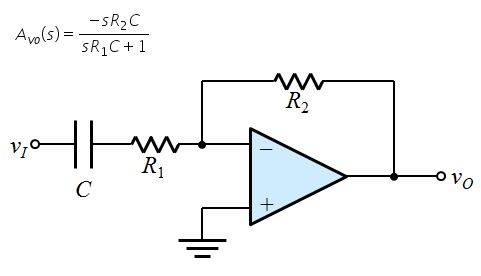# Question For the high-pass filter op-amp circuit below,if R1 = 25kΩ, R2 = 200 kΩ, and C =160 µF, determine the nominal voltage gain of the filter at veryhigh frequencies within the passband,in V/V. Avo(s)= -SR2C SR1C+1 R2 Vio R1 ovo с +HSAHWR The Asker · Electrical Engineering

For the high-pass filter op-amp circuit below, if R1 = 25 kΩ, R2 = 200 kΩ, and C = 160 µF, determine the nominal voltage gain of the filter at very high frequencies within the passband, in V/V.Transcribed Image Text: Avo(s)= -SR2C SR1C+1 R2 Vio R1 ovo с +
More
Transcribed Image Text: Avo(s)= -SR2C SR1C+1 R2 Vio R1 ovo с +